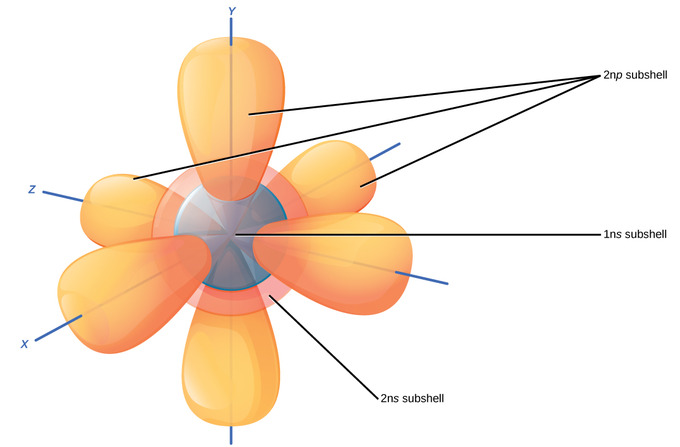# 2.1F: Electron Orbitals

•• Contributed by Boundless
• General Microbiology at Boundless

Electron orbitals are three-dimensional representations of the space in which an electron is likely to be found.

Learning Objectives

• Distinguish between electron orbitals in the Bohr model versus the quantum mechanical orbitals

## Key Points

• The Bohr model of the atom does not accurately reflect how electrons are spatially distributed around the nucleus as they do not circle the nucleus like the earth orbits the sun.
• The electron orbitals are the result of mathematical equations from quantum mechanics known as wave functions and can predict within a certain level of probability where an electron might be at any given time.
• The number and type of orbitals increases with increasing atomic number, filling in various electron shells.
• The area where an electron is most likely to be found is called its orbital.

## Key Terms

• electron shell: The collective states of all electrons in an atom having the same principal quantum number (visualized as an orbit in which the electrons move).
• orbital: A specification of the energy and probability density of an electron at any point in an atom or molecule.

Although useful to explain the reactivity and chemical bonding of certain elements, the Bohr model of the atom does not accurately reflect how electrons are spatially distributed surrounding the nucleus. They do not circle the nucleus like the earth orbits the sun, but are rather found in electron orbitals. These relatively complex shapes result from the fact that electrons behave not just like particles, but also like waves. Mathematical equations from quantum mechanics known as wave functions can predict within a certain level of probability where an electron might be at any given time. The area where an electron is most likely to be found is called its orbital.

## First Electron Shell

The closest orbital to the nucleus, called the 1s orbital, can hold up to two electrons. This orbital is equivalent to the innermost electron shell of the Bohr model of the atom. It is called the 1s orbital because it is spherical around the nucleus. The 1s orbital is always filled before any other orbital. Hydrogen has one electron; therefore, it has only one spot within the 1s orbital occupied. This is designated as 1s1, where the superscripted 1 refers to the one electron within the 1s orbital. Helium has two electrons; therefore, it can completely fill the 1s orbital with its two electrons. This is designated as 1s2, referring to the two electrons of helium in the 1s orbital. On the periodic table, hydrogen and helium are the only two elements in the first row (period); this is because they are the sole elements to have electrons only in their first shell, the 1s orbital.

## Second Electron Shell

The second electron shell may contain eight electrons. This shell contains another spherical s orbital and three “dumbbell” shaped p orbitals, each of which can hold two electrons. After the 1s orbital is filled, the second electron shell is filled, first filling its 2s orbital and then its three p orbitals. When filling the p orbitals, each takes a single electron; once each p orbital has an electron, a second may be added. Lithium (Li) contains three electrons that occupy the first and second shells. Two electrons fill the 1s orbital, and the third electron then fills the 2s orbital. Its electron configuration is 1s22s1. Neon (Ne), on the other hand, has a total of ten electrons: two are in its innermost 1s orbital, and eight fill its second shell (two each in the 2s and three p orbitals). Thus, it is an inert gas and energetically stable: it rarely forms a chemical bond with other atoms.Figure $$\PageIndex{1}$$: Diagram of the S and P orbitals: The s subshells are shaped like spheres. Both the 1n and 2n principal shells have an s orbital, but the size of the sphere is larger in the 2n orbital. Each sphere is a single orbital. p subshells are made up of three dumbbell-shaped orbitals. Principal shell 2n has a p subshell, but shell 1 does not.

## Third Electron Shell

Larger elements have additional orbitals, making up the third electron shell. Subshells d and f have more complex shapes and contain five and seven orbitals, respectively. Principal shell 3n has s, p, and d subshells and can hold 18 electrons. Principal shell 4n has s, p, d, and f orbitals and can hold 32 electrons. Moving away from the nucleus, the number of electrons and orbitals found in the energy levels increases. Progressing from one atom to the next in the periodic table, the electron structure can be worked out by fitting an extra electron into the next available orbital. While the concepts of electron shells and orbitals are closely related, orbitals provide a more accurate depiction of the electron configuration of an atom because the orbital model specifies the different shapes and special orientations of all the places that electrons may occupy.